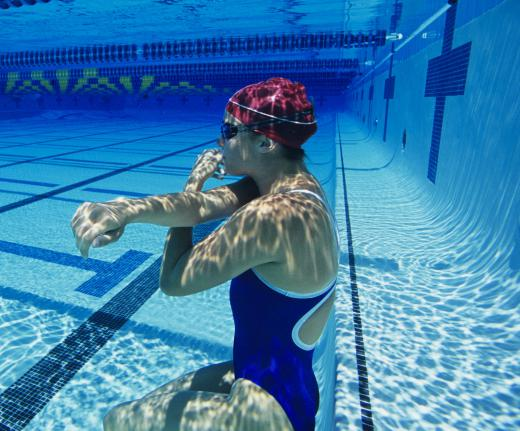# How does a Weighing Tank Work?

R. Stamm
R. StammA person must be completely submerged underwater for a weighing tank to work.

A weighing tank, or hydrostatic weighing tank, works by using Archimedes' principle to determine the density of a person's body and then calculate the percentage of fat in the body. After being weighed normally outside of the hydrostatic weighing tank, the subject sits in a specialized seat and is lowered into the water-filled tank until he or she is completely submerged. The person's underwater weight is then recorded. It is important that the person breathes out as much air as possible before being submerged so that the air in his or her lungs does not create additional buoyancy. The process typically is repeated several time to get an accurate measure of the subject's underwater weight, which is one of the pieces of information used to calculate his or her percentage of body fat.Weighing tanks provide an accurate method of measuring the composition of the body.

Calculating the percentage of fat in the body has a lot to do with the density of each body part in relation to the density of the water. Fat is less dense and has more volume than the skeleton and muscles. It is also less dense than water. Therefore, fat placed into a tank of water should float, because fat is less dense than water.

The skeleton and muscles are denser, with less volume than water and fat. Muscles and the skeleton placed into a tank of water should sink because it denser than water. In other words, when a body is submerged into a weighing tank, it will sink more or float more based on the amount of lean tissue or fat. This is based on Archimedes' principle, which says that when an object is placed in water, the weight of the water displaced will be proportional to the weight of the object.

Along with the subject's weight in and out of the water, several other numbers are needed to calculate the density of a person's body and his or her percentage of body fat. These include residual lung volume, the density of the water and an estimation of the amount of air trapped in the gastrointestinal tract. After the body's density is calculated — the absolute maximum is 1.10, meaning that there is no fat, and the minimum is 0.90, meaning that the body is all fat — an equation called the Siri Equation is used to determine the person's body fat percentage. This equation says that to calculate the percentage of body fat, one must divide 495 by the body’s density, then subtract 450. For example, a body density of 1.07 would yield a body fat percentage of 12.6 percent, because 495 divided by 1.07 is 462.6, and 450 less than that number is 12.6.

Using a weighing tank to calculate body fat percentage is an involved process that requires a great deal of space. Therefore, it is primarily available only at research institutions or colleges and universities. The cost to use it can range from \$10 US Dollars to \$75 USD, depending on where it is performed. Provided that it is done correctly and the calculations are accurate, this is the most effective method for measuring the composition of the body.